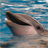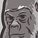# QlikView App Dev

Discussion Board for collaboration related to QlikView App Development.

Announcements
Join this live chat April 6, 10AM EST - QlikView to Qlik Sense REGISTER
cancel
Showing results for
Did you mean:Contributor III

## Calculate decile/quartile/quintile etc when data is aggregated.

Hello,

I've looked through the help and Community pages but can't find a solution to my issue.

I'm attempting to find the decile position of a Course of the average Mark for students on a Course.

Using dummy data with just one value per course it works absolutely perfectly with the following;

if(Mark<=Fractile(TOTAL Mark,0.1),1,

if(Mark<=Fractile(TOTAL Mark,0.2),2,

if(Mark<=Fractile(TOTAL Mark,0.3),3,

if(Mark<=Fractile(TOTAL Mark,0.4),4,

if(Mark<=Fractile(TOTAL Mark,0.5),5,

if(Mark<=Fractile(TOTAL Mark,0.6),6,

if(Mark<=Fractile(TOTAL Mark,0.7),7,

if(Mark<=Fractile(TOTAL Mark,0.8),8,

if(Mark<=Fractile(TOTAL Mark,0.9),9,10)))))))))

In my real data the expression needs to be Avg(Mark) to calculate the average for all students on the course

if(Avg(Mark)<=Fractile(TOTAL Avg(Mark),0.1),1,

if(Avg(Mark)<=Fractile(TOTAL Avg(Mark),0.2),2,

if(Avg(Mark)<=Fractile(TOTAL Avg(Mark),0.3),3,

if(Avg(Mark)<=Fractile(TOTAL Avg(Mark),0.4),4,

if(Avg(Mark)<=Fractile(TOTAL Avg(Mark),0.5),5,

if(Avg(Mark)<=Fractile(TOTAL Avg(Mark),0.6),6,

if(Avg(Mark)<=Fractile(TOTAL Avg(Mark),0.7),7,

if(Avg(Mark)<=Fractile(TOTAL Avg(Mark),0.8),8,

if(Avg(Mark)<=Fractile(TOTAL Avg(Mark),0.9),9,10)))))))))

This gives every Course a value of 10, the final False value above. My thinking so far is that I need to add in Aggr somewhere but everything I've tried has failed.

Any suggestions would be very gratefully received.

John

1 Solution

Accepted SolutionsMVP

May be this

if(Avg(Mark) <= Fractile(TOTAL Aggr(Avg(Mark), Student), 0.1),1,

if(Avg(Mark) <= Fractile(TOTAL Aggr(Avg(Mark), Student), 0.2),2,

if(Avg(Mark) <= Fractile(TOTAL Aggr(Avg(Mark), Student), 0.3),3,

if(Avg(Mark) <= Fractile(TOTAL Aggr(Avg(Mark), Student), 0.4),4,

if(Avg(Mark) <= Fractile(TOTAL Aggr(Avg(Mark), Student), 0.5),5,

if(Avg(Mark) <= Fractile(TOTAL Aggr(Avg(Mark), Student), 0.6),6,

if(Avg(Mark) <= Fractile(TOTAL Aggr(Avg(Mark), Student), 0.7),7,

if(Avg(Mark) <= Fractile(TOTAL Aggr(Avg(Mark), Student), 0.8),8,

if(Avg(Mark) <= Fractile(TOTAL Aggr(Avg(Mark), Student), 0.9),9,10)))))))))

Also, are you using this as an expression or a dimension? If this is an dimension, then try this

Aggr(

if(Avg(Mark) <= Fractile(TOTAL Aggr(Avg(Mark), Student), 0.1),1,

if(Avg(Mark) <= Fractile(TOTAL Aggr(Avg(Mark), Student), 0.2),2,

if(Avg(Mark) <= Fractile(TOTAL Aggr(Avg(Mark), Student), 0.3),3,

if(Avg(Mark) <= Fractile(TOTAL Aggr(Avg(Mark), Student), 0.4),4,

if(Avg(Mark) <= Fractile(TOTAL Aggr(Avg(Mark), Student), 0.5),5,

if(Avg(Mark) <= Fractile(TOTAL Aggr(Avg(Mark), Student), 0.6),6,

if(Avg(Mark) <= Fractile(TOTAL Aggr(Avg(Mark), Student), 0.7),7,

if(Avg(Mark) <= Fractile(TOTAL Aggr(Avg(Mark), Student), 0.8),8,

if(Avg(Mark) <= Fractile(TOTAL Aggr(Avg(Mark), Student), 0.9),9,10)))))))))

, Student)

7 RepliesMVP

May be this

if(Avg(Mark) <= Fractile(TOTAL Aggr(Avg(Mark), Student), 0.1),1,

if(Avg(Mark) <= Fractile(TOTAL Aggr(Avg(Mark), Student), 0.2),2,

if(Avg(Mark) <= Fractile(TOTAL Aggr(Avg(Mark), Student), 0.3),3,

if(Avg(Mark) <= Fractile(TOTAL Aggr(Avg(Mark), Student), 0.4),4,

if(Avg(Mark) <= Fractile(TOTAL Aggr(Avg(Mark), Student), 0.5),5,

if(Avg(Mark) <= Fractile(TOTAL Aggr(Avg(Mark), Student), 0.6),6,

if(Avg(Mark) <= Fractile(TOTAL Aggr(Avg(Mark), Student), 0.7),7,

if(Avg(Mark) <= Fractile(TOTAL Aggr(Avg(Mark), Student), 0.8),8,

if(Avg(Mark) <= Fractile(TOTAL Aggr(Avg(Mark), Student), 0.9),9,10)))))))))

Also, are you using this as an expression or a dimension? If this is an dimension, then try this

Aggr(

if(Avg(Mark) <= Fractile(TOTAL Aggr(Avg(Mark), Student), 0.1),1,

if(Avg(Mark) <= Fractile(TOTAL Aggr(Avg(Mark), Student), 0.2),2,

if(Avg(Mark) <= Fractile(TOTAL Aggr(Avg(Mark), Student), 0.3),3,

if(Avg(Mark) <= Fractile(TOTAL Aggr(Avg(Mark), Student), 0.4),4,

if(Avg(Mark) <= Fractile(TOTAL Aggr(Avg(Mark), Student), 0.5),5,

if(Avg(Mark) <= Fractile(TOTAL Aggr(Avg(Mark), Student), 0.6),6,

if(Avg(Mark) <= Fractile(TOTAL Aggr(Avg(Mark), Student), 0.7),7,

if(Avg(Mark) <= Fractile(TOTAL Aggr(Avg(Mark), Student), 0.8),8,

if(Avg(Mark) <= Fractile(TOTAL Aggr(Avg(Mark), Student), 0.9),9,10)))))))))

, Student)Partner

Hi John using Avg(Total Marks, Course) should help get you towards it I would have thought

or in the script

if(Mark<=Fractile(TOTAL Mark,0.1),1,

if(Mark<=Fractile(TOTAL Mark,0.2),2,

if(Mark<=Fractile(TOTAL Mark,0.3),3,

if(Mark<=Fractile(TOTAL Mark,0.4),4,

if(Mark<=Fractile(TOTAL Mark,0.5),5,

if(Mark<=Fractile(TOTAL Mark,0.6),6,

if(Mark<=Fractile(TOTAL Mark,0.7),7,

if(Mark<=Fractile(TOTAL Mark,0.8),8,

if(Mark<=Fractile(TOTAL Mark,0.9),9,10)))))))));

Resident Data Group by CourseMVP

Preceding load will not work as you are using another aggregation (Fractile) without a group by. Also, I don't think there is such a thing as TOTAL in the scriptPartner

Sorry TOTAL Mark won't work in the script,  but that could be calculated and placed into a variable (or each Fractile could be placed into its own variable then referenced in the scriptMVP

Its going to be slightly more complicated then you are portraying, but it depends on what OP wants to do hereContributor III
Author

Thanks Sunny, I was using it in an expression so went with the first part. It didn't work aggregating at student level but when I substituted in Course it gave me the results I was expecting.

JohnContributor III
Author

Thanks Dave, hopefully this is just a one off so am going with what seems to be the simpler option. I might need to come back to you sometime in the future about adding expressions into variables!

John